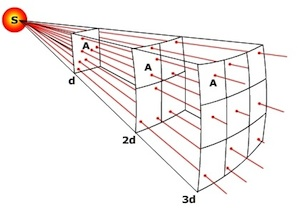$$\newcommand{\vecs}{\overset { \rightharpoonup} {\mathbf{#1}} }$$ $$\newcommand{\vecd}{\overset{-\!-\!\rightharpoonup}{\vphantom{a}\smash {#1}}}$$$$\newcommand{\id}{\mathrm{id}}$$ $$\newcommand{\Span}{\mathrm{span}}$$ $$\newcommand{\kernel}{\mathrm{null}\,}$$ $$\newcommand{\range}{\mathrm{range}\,}$$ $$\newcommand{\RealPart}{\mathrm{Re}}$$ $$\newcommand{\ImaginaryPart}{\mathrm{Im}}$$ $$\newcommand{\Argument}{\mathrm{Arg}}$$ $$\newcommand{\norm}{\| #1 \|}$$ $$\newcommand{\inner}{\langle #1, #2 \rangle}$$ $$\newcommand{\Span}{\mathrm{span}}$$ $$\newcommand{\id}{\mathrm{id}}$$ $$\newcommand{\Span}{\mathrm{span}}$$ $$\newcommand{\kernel}{\mathrm{null}\,}$$ $$\newcommand{\range}{\mathrm{range}\,}$$ $$\newcommand{\RealPart}{\mathrm{Re}}$$ $$\newcommand{\ImaginaryPart}{\mathrm{Im}}$$ $$\newcommand{\Argument}{\mathrm{Arg}}$$ $$\newcommand{\norm}{\| #1 \|}$$ $$\newcommand{\inner}{\langle #1, #2 \rangle}$$ $$\newcommand{\Span}{\mathrm{span}}$$$$\newcommand{\AA}{\unicode[.8,0]{x212B}}$$

It's hard to imagine radiation moving as waves through empty space without a medium to transfer the wave form. For instance, the waves created when you drop a rock into a pool of water require molecules of H2O to propagate them. Though we describe electromagnetic radiation as invisible waves of energy, at the smallest scale it behaves as a particle, like when light is emitted by a single atom or molecule. When energy is given off there is a change in the orbital pattern of the electrons that surround the nucleus of an atom. As the orbit changes, a bundle of energy called a "photon" is released. However, particles of light differ from particles of matter: they have no mass, occupy no space, and travel at the speed of light, 2.9998 X 108 m s-1. The amount of energy carried by a photon varies inversely with wavelength, the shorter the wavelength, the more energetic the photon.

In order to understand the processes and explain patterns of weather and climate, it is helpful to dig a bit deeper into a few laws related to energy and radiation.

Stefan-Boltzmann's Law: The total energy emitted by a black body is proportional the 4th power of its absolute temperature. Substances that emit the maximum amount of radiation for their temperature in all wavelengths are called black bodies. Also known as Stefan's law, it can be expressed as

$E\,=\sigma T^4$

where Ε is energy emitted per second from a unit are of a black body with a temperature of T(in Kelvin) and $$\sigma$$ is the Stefan-Bloltzmann constant of 5.670 X 10-8J K-4 m-2 s-1

Wein's Law: The hotter the substance, the shorter the wavelength of emission. Wein's Law, introduced earlier in this chapter can be expressed as

$\lambda_{(max)}\,=\,\frac{a}{T}$

where a is the constant = 2897 μm K and T is temperature (in Kelvin). Using Wein's law reveals why the Sun emits most of its radiation as shortwave and the earth as longwave. Using average surface temperatures for the Sun and Earth

Sun: $$\lambda_{(max)}\,=\,\frac{2897\mu m\,K}{5778\,K}\,=\,0.50\,\mu m$$

Earth: $$\lambda_{(max)}\,=\,\frac{2897\mu m\,K}{287\,K}\,=\,10\,\mu m$$

Hence, the Sun emits most of its energy in the shortwave end of the electromagnetic spectrum while the Earth emits most of its energy in the longwave end.

Inverse Square LawThe intensity of light emanating from a point source is inversely proportional to the square of the distance from the source. The inverse square law explains why the intensity of radiation received diminishes as one moves away from the source. Notice how light (radiation) spreads as you move away from point S. Imagine a light sensor placed in the path of the light at distance d and measuring the intensity of light on one unit of area (A). As we move further away from the source, the light continues to spread, though our sensor still measures the light hitting one unit of area. When we reach a distance of 2d, the light now has spread to 4 units of area, but only 1/4 of the light falls on the sensor. If we move to 3 times the distance (3d) the light spreads further and now 1/9 of the light falls on the sensor.Figure $$\PageIndex{1}$$: The Inverse Square Law illustrated.

The diminishing intensity of light as one moves away from the source is expressed mathematically as,

$S_d\,=\,S_0\,(\frac{d_0}{d})^2$

where S is the solar intensity at some distance d, and S0 is the intensity at some reference distance d0.

Kirchoff's Law: Good absorbers are good emitters at a particular wavelength, and poor absorbers are poor emitters at the same wavelengths. In other words, some things, like the gases of Earth's atmosphere, are selective absorbers and emitters. Kirchoff's law helps explain phenomena like the greenhouse effect and why the warmest evening occur when it is cloudy. Carbon dioxide is a good absorber and emitter of of infrared radiation and thus plays an important role in the greenhouse effect. Clouds are also good absorbers and emitters of infrared radiation, creating warmer conditions than cloudless conditions during the night.

This page titled 4.1.2: Radiation as Particles is shared under a CC BY-SA 4.0 license and was authored, remixed, and/or curated by Michael E. Ritter (The Physical Environment) via source content that was edited to the style and standards of the LibreTexts platform; a detailed edit history is available upon request.